Related Articles

# Equation of a Circle

Equation of a circle is the algebraic way to define a circle. It is the locus of the point which is at a constant distance from a fixed point. The fixed point is called the centre of the circle, and the constant distance is called the radius of the circle. The equation of a circle is very useful for finding different parameters such as the circumference, and area of the circle.

A circle in 2-D and the 3-D planes is represented using the equation of the circle. It is the algebraic equation solution of which represents all the points on the circumference of the circle. We can represent the equation of the circle in various forms, some of the common forms of the equation of the circle are,

• General form
• Standard form
• Parametric form
• Polar form

In this article, let’s learn about the equation of the circle, and all its various forms and others.

## What is Equation of Circle?

The equation of a circle is a way to represent the curve of a circle in the 2-D and the 3-D plane. It is the locus of the point which represents the path of the point which is at a fixed distance from a fixed point. The algebraic equation of the circle can be found using the coordinate of the centre and the length of the radius of the circle, where the centre of the circle is the fixed point from which the radius is calculated.

In general, if the centre of the circle is represented with (h, k) and the radius of the circle is ‘r’ then the equation of the circle is,

(x-h)2 + (y-k)2 = r2

This equation represents all the points that are at r distance from the point (h, k) in the 2-D coordinate plane.

## Different Forms of Equation of Circle

We can represent the equation of a circle using various forms. In each form, a different set of values is given which is used to form the equation of the circle. All these forms can represent the same circle, but their initial parameters are different. In all these forms of equations of a circle, we can easily find the radius and centre of the circle.

Various forms to represent the equation of a circle are,

• General form
• Standard form
• Parametric form
• Polar form

## General Equation of a Circle

The general form of the equation of a circle is represented as,

x2 + y2 + 2gx + 2fy + c = 0

where,
g, f, and c are contsnat values

Using this general form of the equation we can easily find the centre and radius of the circle. For, finding the centre and the radius of the circle we use the completing the square method and then compare it with the standard form.

Converting the general form of the equation x2 + y2 + 2gx + 2fy + c = 0, into the standard form

x2 + y2 + 2gx + 2fy + c = 0

Adding f2, g2, and -c on both sides,

x2 + y2 + 2gx + 2fy + c + f2 + g2 -c = f2 + g2 -c

x2 + 2gx + g2 + y2 + 2fy + f2 = f2 + g2 -c

(x + g)2 + (y – f)2 = [√(f2 = f2 + g2 -c)]2

comparing with (x-h)2 + (y-k)2 = r2

we get,

• centre = (h, k) = (-g, -f)
• radius = r = √(f2 = f2 + g2 -c)

### Features of General Equation of Circle

x2 + y2 + 2gx + 2fy + c = 0, represents the general equation of a circle with centre (−g, −f) and radius (r): r2 = g2 + f2− c. Some of the important results which we deduce from the general equation of the circle are,

• If g2 + f2 > c, then the radius of the circle is real.
• If g2 + f2 = c, then the radius of the circle is zero which tells us that the circle is a point which coincides with the centre. Such type of circle is called a point circle
• g2 + f2 <c, then the radius of the circle becomes imaginary. Therefore, it is a circle having a real centre and imaginary radius.

## Standard Equation of a Circle

The standard equation of the circle is also the basic equation of the circle. It is the most common equation of a circle and is widely used. It gives the centre and radius of the circle in the easiest and most efficient way. The standard form of the equation of the circle is

(x-h)2 + (y-k)2 = r2

where, (h, k) represents the centre of the circle and r represents the radius of the circle. Here, (x, y) are any arbitrary point on the circumference of the circle from which the centre of the circle is at the constant distance.

To find the standard form of the circle let us assume that A(h, k) is the centre of the circle and r is the radius of the circle and P(x, y) is any point on the circumference.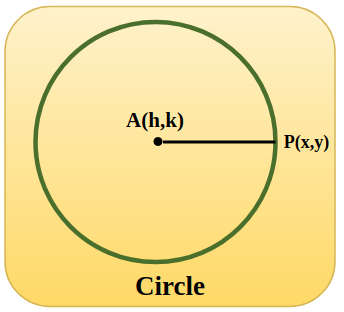Then, AP = r …..(i)

Now using the distance formula we find the value of the AP

AP =Now put the value of AP in eq(i), and we get= r

Or squaring both sides we get

(x – h)2 + (y – k)2 = r

This is the standard form of the equation of the circle.

Example: If the centre and the radius of the circle are (3, 5) and 7 units respectively then find the equation of the circle.

Solution:

Centre of the circle is (3, 5) comparing with (h, k) we get h = 3 and k = 5

Similarly, radius of the circle is 7 = r

Equation of the circle is (x – h)2 + (y – k)2 = r

(x – 3)2 + (y – 5)2 = 7

This is the required equation of the circle.

## Parametric Equation of a Circle

The parametric form of the circle uses (-h + rcosθ, -k + rsinθ) as the general point on the circumference of the circle. The line joining the centre of the circle to the general point makes an angle θ with the x-axis. Thus, the parametric equation of a circle is

x2 + y2 + 2hx + 2ky + c = 0

General point on the circumference of the circle is  (-h + rcosθ, -k + rsinθ).

## Polar Equation of a Circle

The other form of the equation of the circle is the polar form of the circle, this form of the circle is similar to the parametric form of the circle.

Suppose we take a point on the circumference of the circle (x, y) as (acosθ, bsinθ) when the line joining the point with the centre of the circle makes an angle θ with the x-axis and the radius of the circle is ‘a’. Also, the centre of the circle is the origin, (0,0) then the equation of the circle is,

x = a cosθ…(i) and y = a sinθ…(ii)

Now we can easily convert the above equation into the standard form of the circle as,

Squaring and adding eq(i) and eq(ii) we get,

x2 + y2 = a2 cos2θ + a2 sin2 θ

x2 + y2 = a2(cos2θ + sin2 θ)

Using Trigonometric Identity, cos2θ + sin2 θ  =1

x2 + y2 = a2

(x-0)2 + (y-0)2 = a2 comparing with (x – h)2 + (y – k)2 = r we get

• Centre = (h, k) = (0, 0)
• Radius = r = a

Example: Find the equation of the circle in the polar if the equation of the circle in standard form is x2 + y2 = 16.

Solution:

Equation of the circle in polar form is,

x = r cosθ

y = r sinθ

using this in x2 + y2 = 16

(r cosθ)2  + (r sinθ)2 = 16

r2(cos2θ + sin2θ) = 16

r2 = 42

r = 4

## Equation of a Circle Formula

The formula used to calculate the equation of the circle is,

(x – x1)2 + (y – y1)2 = r2,

where, (x1, y1) is the centre of the circle and r is the radius of the circle.

This is the basic formula for finding the equation of the circle. We use this formula to easily find the equation of the circle, for example, find the equation of the circle if the centre of the circle is (1, 2) and its radius is 5 units,

Solution:

Given, centre = (1, 2) and radius = 5 units

Equation of the circle fromula is,

(x – x1)2 + (y – y1)2 = r2

(x -1)2 + (y – 2)2 = 52

x2 + 1 – 2x + y2 + 4 – 4y = 25

x2 + y2 – 2x – 4y + 5 = 25

x2 + y2 – 2x – 4y – 20 = 0

This is the general equation of the circle.

## Derivation of Circle Equation

The equation of the circle is easily derived using the distance formula,

Suppose we take a circle with centre (a, b) and radius r then any general point the circumference of the circle is taken as (x, y).

We know that distance between the point (x, y) and the centre (a, b) is given using the distance formula, also the distance between (a, b) and the (x, y) is the radius of the circle, i.e.

Distance between (x, y) and (a, b) = Radius of the Circle

√[(x -a)2 + (y – b)2] = r

Squaring both sides,

[(x -a)2 + (y – b)2] = r2

This is the required equation of the circle.

## Graphing Equation of Circle

As we know that the standard equation of the circle is

(x – h)2 + (y – k)2 = r

Here, the centre of the circle is (h, k) and the radius of the circle is r. Now let us take an example,

The equation of the circle is (x + 2)2 + (y – 6)2 = 4

we can rewrite this equation as

(x – (-2))2 + (y – 6)2 = 22

On comparing with the standard equation of the circle we get

The centre of the circle is (-2, 6) and the radius of the circle is 2.

Now we will draw a circle on the graph.

Step 1: Draw the x and y-axis

Step 2: Plot the centre of the circle on the graph that is (-2, 6)

Step 3: Take the radius of 2 units in the compass and draw the required circle.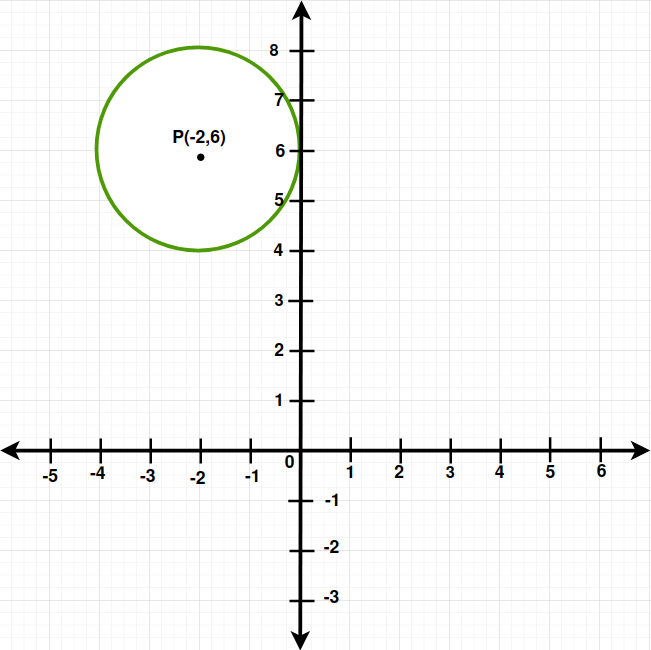## How to Find Equation of Circle?

Equation of the circle can be easily found using the various parameters as given in the questions. If we are given the conditions to find the centre and the radius of the circle then its cartesian equation can easily be written. We will learn to write the equation of a circle under the following conditions:

• Equation of Circle when centre at (x1,y1)
• Equation of Circle with Center at the origin
• Equation of Circle with Centre on X-axis
• Equation of Circle when Centre on Y- axis
• Equation of Circle touching X-axis
• Equation of Circle touching Y-axis
• Equation of Circle touching both axes

Now, we can easily write the equations of the circle using the initial condition. Let’s learn about the different conditions for writing the equation of the circle.

## Equation of Circle when Center at (x1, y1)

The equation of the circle when the centre of the circle is at (x1, y1) and its radius is ‘r'(let) is given using the following steps,

Step 1: Note the centre (x1, y1) and the radius ‘r’ of the circle.

Step 2: Use the Equation of the circle formula,

(x – x1)2 + (y – y1)2 = r2

Step 3: Substitute the value of centre (x1, y1) and radius ‘r’.

Step 4: Simplify the above equation to find the required equation of the circle.

## Equation of Circle With Center at the Origin

The equation of the circle when the centre of the circle is at origin i.e. (0, 0) and its radius is ‘r'(let) is given using the following steps,

Step 1: Note the centre (0, 0) and the radius ‘r’ of the circle.

Step 2: Use the Equation of the circle formula,

(x – x1)2 + (y – y1)2 = r2

Step 3: Substitute the value of centre (0, 0) and radius ‘r’.

Step 4: Simplify the above equation to find the required equation of the circle.

(x – 0)2 + (y – 0)2 = r2

x2 + y2 = r2

This is the required equation of the circle with the centre origin and radius ‘r’.

## Equation of Circle with Centre on x-axis

We know that the general point on the x-axis is (a, 0). Suppose we have to find the equation of the circle with centre at the x-axis i.e. (a, 0) and its radius is ‘r’ unit. Then its equation is given by substituting the value of the centre and radius in,

(x – x1)2 + (y – y1)2 = r2

(x – a)2 + (y – 0)2 = r2

(x – a)2 + y2 = r2

This is the required equation of a circle with a centre on the x-axis.

## Equation of Circle with Centre on y-axis

We know that the general point on the y-axis is (0, b). Suppose we have to find the equation of the circle with the centre at the y-axis i.e. (0, b) and its radius is ‘r’ unit. Then its equation is given by substituting the value of the centre and radius in,

(x – x1)2 + (y – y1)2 = r2

(x – 0)2 + (y – b)2 = r2

x2 + (y – b)2 = r2

This is the required equation of a circle with a centre on the y-axis.

## Equation of Circle Touching x-axis

We can easily find the equation of a circle touching the x-axis. Suppose the circumference of the circle touches the x-axis at point (a, 0) and (a, r) is the centre of the circle with radius r.

We know that if a circle touches the x-axis, then its y-coordinate of the centre of the circle is equal to the radius r, this condition is shown in the image below,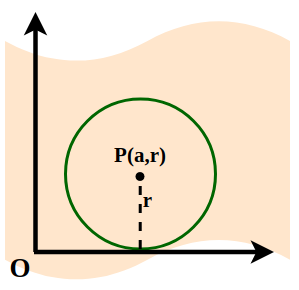Take any general point (x, y) on the circumference of the circle then its equation is given as,

Distance between (x, y) and (a, r) = Radius(r)

√[(x -a)2 + (y-r)2] = r

Squaring both sides we get,

(x -a)2 + (y-r)2 = r2

This is the equation of the circle touching the x-axis.

## Equation of Circle Touching y-axis

We can easily find the equation of a circle touching the y-axis. Suppose the circumference of the circle touches the y-axis at point (0, b) and (r, b) is the centre of the circle with radius r.

We know that if a circle touches the y-axis, then its x-coordinate of the centre of the circle is equal to the radius r, this condition is shown in the image below,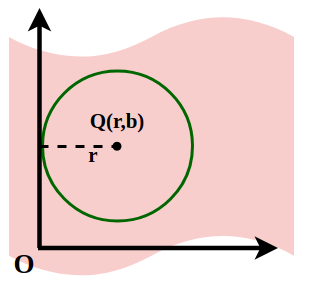Take any general point (x, y) on the circumference of the circle then its equation is given as,

Distance between (x, y) and (r, b) = Radius(r)

√[(x -r)2 + (y-b)2] = r

Squaring both sides we get,

(x -r)2 + (y-b)2 = r2

This is the equation of the circle touching the y-axis.

## Equation of Circle Touching both axes

We can easily find the equation of a circle touching both the axis i.e. x-axis, and the y-axis. Suppose the circumference of the circle touches the x-axis at point (r, 0)and the y-axis at point (0, r) and (r, r) is the centre of the circle with radius r.

We know that if a circle touches both x and y-axis, then its x-coordinate and y-coordinate of the centre of the circle are equal to the radius r, this condition is shown in the image below,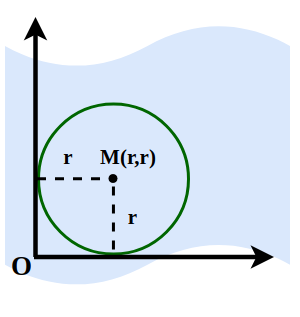Take any general point (x, y) on the circumference of the circle then its equation is given as,

Distance between (x, y) and (r, r) = Radius(r)

√[(x -r)2 + (y-r)2] = r

Squaring both side we get,

(x -r)2 + (y-r)2 = r2

This is the equation of the circle touching both the axes i.e. x-axis and y-axis.

## Converting General Form to Standard Form

The general form of the circle i.e. x2 + y2 + 2gx + 2fy + c = 0 can be easily converted to the standard form of the circle i.e. (x – a)2 + (y – b)2 = r2 using the steps discussed below,

Step 1: Write the like terms together and take the constant on the other side of the equation to as x2 + 2gx + y2 + 2fy = – c…(i)

Step 2: Use completing the square method simplify the left-hand side of the ‘equal to’ by adding g2, and f2 on both sides.

x2 + 2gx + y2 + 2fy + g2 + f2 = – c + g2 + f2

Step 3: Simplify the above equation.

x2 + 2gx + g2 + y2 + 2fy + f2 = g2 + f2 – c

(x+g)2 + (y-f)2 = [√(g2 + f2 – c)]2

Comparing the above equation with the standard equation of the circle as, (x – a)2 + (y – b)2 = r2 we get,

• Center = (-g, -f)
• Radius = √(g2 + f2 – c)

Thus, by using the above method general form of the is transformed into the standard form of the equation.

## Converting Standard Form to General Form

The standard form of the circle i.e. (x – a)2 + (y – b)2 = r2  can be easily converted to the general form of the circle i.e. x2 + y2 + 2gx + 2fy + c = 0  using the steps discussed below,

Step 1: Use the algebraic identity formula, (a-b)2 = a2 + b2 -2ab to simplify the given equation (x – a)2 + (y – b)2 = r2

x2 + a2 – 2ax + y2 + b2 – 2by = r2

Step 2: Arrange the equation with variable terms together and constant together as,

x2 – 2ax + y2 – 2by + a2 + b2 – r2 = 0

Comparing with x2 + y2 + 2gx + 2fy + c = 0 we get,

g = -a, f = -b, and c = a2 + b2 – r2

This is the required form of the equation of a circle in a general form.

## Features of Equation of Circle

The general form of the equation of the circle is x2 + y2 + 2gx + 2fy + c = 0. Some of the features of the equation of the circle are,

• It is quadratic in both x and y.
• Coefficient of x2 = y2. (It is advisable to keep the coefficient of x2 and y2 unity)
• There is no term containing xy i.e., the coefficient of xy is zero.
• It contains three arbitrary constants viz. g, f and c

## Examples on Circle Equations

Example 1: Find the centre and radius of the circle from the given equation:  x2 + y2 + 6x – 4y + 4 = 0

Solution:

We have,

(x2 + 6x) + (y2 – 4y) = – 4

To make it a perfect square identity, add and subtract 9 and 4,

(x2 + 6x + 9) + (y2 – 4y + 4) – 9 – 4 = – 4

(x + 3)2 + (y – 2)2 = – 4 + 4 + 9

(x – (-3))2 + (y – 2)2 = 9

(x – (-3))2 + (y – 2)2 =32

On comparing with the standard equation of circle, we have

h = -3, k = 2 and r = 3

So the centre of the circle is (-3, 2)

and the radius of the circle = 3

Example 2: Find the equation of a circle when the endpoints of the diameter are (3, 2) and (5, 8).

Solution:

Given,

End points of the diameter are (3, 2) and (5, 8)

As we know radius is the mid point of the diameter then,

The coordinate of the radius are, [(3+5)/2, (2+8)/2] = (4, 5)

Length of radius = √[(4-3)2 + (5-2)2] = √(1+9)

= √(10)

Equation of the circle is,

(x-a)2 + (y-b)2 = r2

where, (a, b) is centre and r is the radius,

Now,

(x-4)2 + (y-5)2 = [√(10)]2

The required equation of the circle is (x-4)2 + (y-5)2 = 10 whose end points of the diameter are (3, 2) and (5, 8).

Example 3: Find the centre and radius of the circle using the following equation: x2 + y2 – 4x + 6y = 12.

Solution:

Given: x2 + y2 – 4x + 6y = 12

We can write as

(x2 – 4x) + (y2 – 6y) = 12

By manipulating identity, we get

(x2 – 4x + 4) + (y2 6y + 9) – 9 – 4 = 12

(x2 – 4x + 4) + (y2 – 6y + 9) = 12 + 9 + 4

(x – 2)2 + (y – 3)2 = 25

(x – 2)2 + (y – 3)2 = 52

On comparing with the standard equation of circle, we have

h = 2, k = 3 and r = 5.

Example 4: Find the equation of a circle whose centre is (-3, -2) and whose radius is 6.

Solution:

Given,

h = -3, k = -2, and, r = 6

Using the standard equation of circle,

(x – h)2 + (y – k)2 = r

(x – (-3))2 + (y – (-2))2 = 62

(x + 3)2 + (y + 2)2 = 62

x2 + 6x + 9 + y2 + 4y + 4 = 36

Hence, the required equation is

x2 + y2 + 6x + 4y – 23 = 0

Example 5: Find the equation of a circle whose radius is 7 and the centre is at the origin.

Solution:

Given : r = 7 and the centre = (0, 0)

Using the standard equation of circle,

(x – h)2 + (y – k)2 = r

x2 + y2 = r2

x2 + y2 = 72

Hence, the required equation is

x2 + y2 – 49 = 0

Example 6: Find the equation of the circle that passed through the points (1, 0), (-1, 0), and (0, 1).

Solution:

Let the required circle be

x2 + y2 + 2gx + 2fy + c = 0

As, it passes through (1, 0), (-1, 0), and (0, 1). Hence, they will satisfy the equation

By substituting them, we get

1 + 2g + c = 0 …(1)

1 – 2g + c = 0 …(2)

1 + 2f + c = 0 …(3)

From eq(1) and (2), we get

g = 0 and c = -1

Now, putting c = -1 in eq(3), we get

f = 0

Now, substituting the values of g, f and c in the main equation, we get

x2 + y2 = 1

Example 7: Find the equation of the image of the circle x2 + y2 + 16x – 8y + 64 = 0 in the line mirror x = 0.

Solution:

Given equation is,

x2 + y2 + 16x – 8y = – 64

(x2 + 16x) + (y2 – 8y) = – 64

On adding and subtracting 64 and 16, we get

(x2 + 16x + 64) + (y2 – 8y + 16) – 64 – 16 = – 64

(x2 + 16x + 64) + (y2 – 8y + 16) = – 64 + 64 + 16

(x + 8)2 + (y – 4)2 = 16

(x – (-8))2 + (y – 4)2 = 42

As, the radius of this circle is (-8, 4) and radius = 4.

The image of the circle in the line mirror will have its centre as (8, 4) and radius 4.

So, the equation will be,

(x – 8)2 + (y – 4)2 = 42

x2 + y2 – 16x – 8y + 64 = 0

## FAQs on Equation of a Circle

### Q1: What is the Equation for a Circle?

The standard equation for a circle is,

(x-h)2+(y-k)2 = r2

where
(h, k) is the center,
r‘ is the radius of the circle.

### Q2: What is the General Equation of Circle?

The general equation of the circle is, x2 + y2 + 2gx + 2fy + c = 0

### Q3: What is the Parametric Equation of Circle?

Parametric Equation of the circle is, x2 + y2 + 2hx + 2ky + c = 0 where x = -h + rcosθ and y = -k + rsinθ

### Q4: What is the Equation of Circle in Polar form?

The equation of circle in polar form is, x2 + y2 = a2

• x = a cosθ
• y = a sinθ

where,
a is the radius of the circle

### Q5: What is the Equation of Point Circle?

A point circle is the circle whose radius is zero. The equation of the point circle is,

(x-a)2 + (y-b)2 = 0

where, (a, b) is the radius of the circle.

### Q6: What are the Formulas for Circles?

Various formulas for the circle are,

• Circumference of a Circle = 2πr
• Area of a Circle = πr2

where,
π is the constant and its value is 3.1415,
r is the radius of the circle.

### Q7: What is the Equation of a Circle when the Centre is at the Origin?

When the centre of the circle is at origin (0,0) its equation is given as, (x -0)2 + (y-0)2 = a2

x2 + y2 = a2

where a is the radius of the circle.

Whether you're preparing for your first job interview or aiming to upskill in this ever-evolving tech landscape, GeeksforGeeks Courses are your key to success. We provide top-quality content at affordable prices, all geared towards accelerating your growth in a time-bound manner. Join the millions we've already empowered, and we're here to do the same for you. Don't miss out - check it out now!

Previous
Next